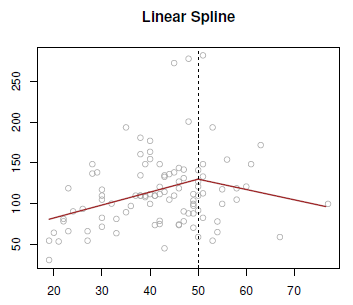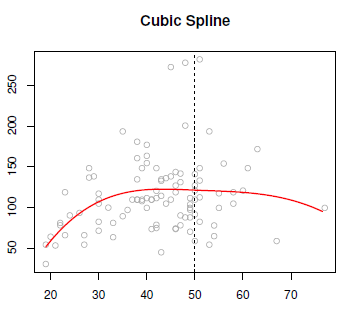# Statistics - Power of a test

The power of a test sometimes, less formally, refers to the probability of rejecting the null when it is not correct, the chance that your experiment is right.

A test's power is influenced by the choice of significance level for the test, the size of the effect being measured, and the amount of data available.

A hypothesis test may fail to reject the null, for example, if a true difference exists between two populations being compared by a t-test but the effect is small and the sample size is too small to distinguish the effect from random chance.

## Example

Many clinical trials, for instance, have low statistical power to detect differences in adverse effects of treatments, since such effects may be rare and the number of affected patients small.

## Documentation / Reference

Recommended PagesStatistics - (Case-control|retrospective) sampling

Statistics - (Case-control|retrospective) sampling The case-control sampling comes in a study in which subjects arenot randomized to exposed or unexposed groups, but rather the subjects are observed...Statistics - (Linear spline|Piecewise linear function)

A linear spline, or piecewise linear function has a degree zero and is: linear in the left and the right. forced to be continuous at the knot. Just like the global polynomials and the piecewise...Statistics - Cubic spline

Cubic splines is a step cubic piecewise polynomial in the left and the right and continuous at the knot. There's the following constraints: The first derivatives continues at the knot, and the...